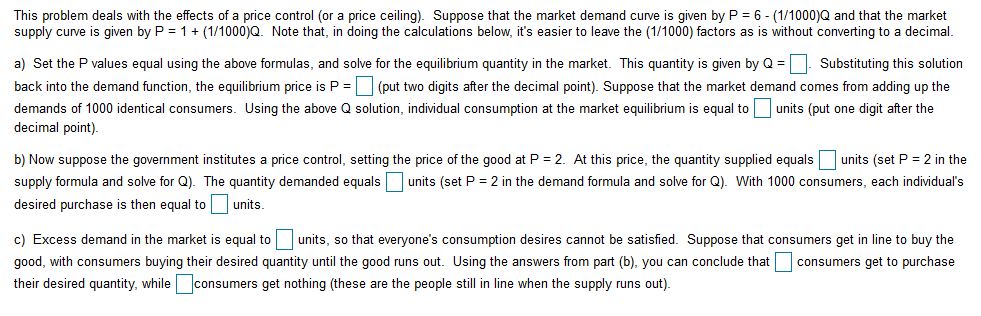# This problem deals with the effects of a price control (or a price ceiling). Suppose that the market demand curve is given by P = 6- (1/1000)Q and that the marketsupply curve is given by P 1(1/1000)Q. Note that, in doing the calculations below, it's easier to leave the (1/1000) factors as is without converting to a decimalSubstituting this solutionback into the demand function, the equilibrium price is P (put two digits after the decimal point). Suppose that the market demand comes from adding up thea) Set the P values equal using the above formulas, and solve for the equilibrium quantity in the market. This quantity is given by Qdemands of 1000 identical consumers. Using the above Q solution, individual consumption at the market equilibrium is equal tounits (put one digit after thedecimal point)b) Now suppose the government institutes a price control, setting the price of the good at P 2. At this price, the quantity supplied equalsunits (set P 2 in thesupply formula and solve for Q). The quantity demanded equals units (set P 2 in the demand formula and solve for Q). With 1000 consumers, each individual'sdesired purchase is then equal tounits.units, so that everyone's consumption desires cannot be satisfied. Suppose that consumers get in line to buy thec) Excess demand in the market is equal togood, with consumers buying their desired quantity until the good runs out. Using the answers from part (b), you can conclude thatconsumers get to purchaseconsumers get nothing (these are the people still in line when the supply runs out)their desired quantity, while

Question
23 viewshelp_outlineImage TranscriptioncloseThis problem deals with the effects of a price control (or a price ceiling). Suppose that the market demand curve is given by P = 6- (1/1000)Q and that the market supply curve is given by P 1(1/1000)Q. Note that, in doing the calculations below, it's easier to leave the (1/1000) factors as is without converting to a decimal Substituting this solution back into the demand function, the equilibrium price is P (put two digits after the decimal point). Suppose that the market demand comes from adding up the a) Set the P values equal using the above formulas, and solve for the equilibrium quantity in the market. This quantity is given by Q demands of 1000 identical consumers. Using the above Q solution, individual consumption at the market equilibrium is equal tounits (put one digit after the decimal point) b) Now suppose the government institutes a price control, setting the price of the good at P 2. At this price, the quantity supplied equalsunits (set P 2 in the supply formula and solve for Q). The quantity demanded equals units (set P 2 in the demand formula and solve for Q). With 1000 consumers, each individual's desired purchase is then equal to units. units, so that everyone's consumption desires cannot be satisfied. Suppose that consumers get in line to buy the c) Excess demand in the market is equal to good, with consumers buying their desired quantity until the good runs out. Using the answers from part (b), you can conclude that consumers get to purchase consumers get nothing (these are the people still in line when the supply runs out) their desired quantity, while fullscreen
check_circle

Step 1

Demand function is given:

Step 2

a) The equilibrium quantity can be calculated by equating the above functions.

Step 3

Hence, the equilibrium quantity is 2500 units.

Substituting the q...

### Want to see the full answer?

See Solution

#### Want to see this answer and more?

Solutions are written by subject experts who are available 24/7. Questions are typically answered within 1 hour.*

See Solution
*Response times may vary by subject and question.
Tagged in